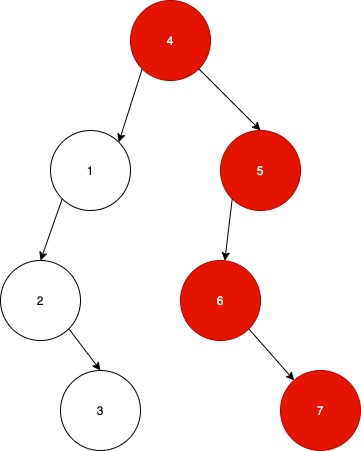# Longest Consecutive Sequence In A Binary Tree

Posted: 13 Dec, 2020
Difficulty: Moderate

## PROBLEM STATEMENT

#### For example:#### Note that there is another consecutive sequence of length 3 i.e. 1->2->3 in the left subtree of the root node, but it is not the longest consecutive path.

##### Input Format :
``````The first line contains an Integer 'T' which denotes the number of test cases or queries to be run. Then the test cases follow.

The first line of input contains the elements of the tree in the level order form separated by a single space.

If any node does not have left or right child, take -1 in its place. Refer to the example below.

Example :

Elements are in the level order form. The input consists of values of nodes separated by a single space in a single line. In case a node is null, we take -1 on its place.

For example, the input for the tree depicted in the below image would be :
````````````1
2 3
4 -1 5 6
-1 7 -1 -1 -1 -1
-1 -1

Explanation :
Level 1 :
The root node of the tree is 1

Level 2 :
Left child of 1 = 2
Right child of 1 = 3

Level 3 :
Left child of 2 = 4
Right child of 2 = null (-1)
Left child of 3 = 5
Right child of 3 = 6

Level 4 :
Left child of 4 = null (-1)
Right child of 4 = 7
Left child of 5 = null (-1)
Right child of 5 = null (-1)
Left child of 6 = null (-1)
Right child of 6 = null (-1)

Level 5 :
Left child of 7 = null (-1)
Right child of 7 = null (-1)

The first not-null node (of the previous level) is treated as the parent of the first two nodes of the current level. The second not-null node (of the previous level) is treated as the parent node for the next two nodes of the current level and so on.

The input ends when all nodes at the last level are null (-1).
``````
##### Note :
``````The above format was just to provide clarity on how the input is formed for a given tree.

The sequence will be put together in a single line separated by a single space. Hence, for the above-depicted tree, the input will be given as:

1 2 3 4 -1 5 6 -1 7 -1 -1 -1 -1 -1 -1
``````
##### Output Format :
``````For each test case, return a single integer denoting the number of nodes in the  Longest increasing Consecutive Path.
``````
##### Note
``````Do not print anything, it has already been taken care of. Just implement the given function.
``````
##### Constraints :
``````1 <= T <= 100
1 <= N <= 3000

Where ‘N’ is the number of nodes in the given binary tree.

Time Limit: 1 sec
``````Approach 1
• The idea is to traverse the binary tree and for each node check if any of its children is the successor of the parent.
• If yes, we will increment the path length.
• We recursively traverse the tree and return the greatest path length found.

Keeping in mind the above idea, we can devise the following recursive  approach:

• We start our recursive function with the parameters ‘root’ which is the root node of the tree, ‘currentLength’ which is the length of the path in the current recursive call, ‘expected’ which stores the expected value for the node, it wil be equal to the value of parent +1 for any node and is initialised with the value in the root node.
• If the root node is null we just return 0.
• Otherwise recursively check both left and right subtree if the value in any of the children is the successor of the root node increment the ‘currentLength’ and compare it with the global maximum value.
• Finally, return the global maximum value.